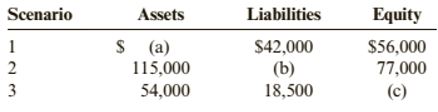Chapter 1, Problem 14CE### Cornerstones of Financial Accounti...

4th Edition
Jay Rich + 1 other
ISBN: 9781337690881

#### Solutions

Chapter
Section### Cornerstones of Financial Accounti...

4th Edition
Jay Rich + 1 other
ISBN: 9781337690881
Textbook Problem
44 views

# Cornerstone Exercise 1-14Using the Accounting EquationListed below are three independent scenarios.Required:Use the fundamental accounting equation to find the missing amounts.

To determine

Concept Introduction:

Accounting equation:

Accounting equation represents the mathematical relationship between assets, liabilities and equity. According to this equation, assets are equal to the sum of liabilities and equity. The formal for basic accounting equation is as follows:

Assets = Liabilities + Equity

To Calculate:

The missing amounts using the accounting equation.

Explanation

The missing amounts are calculated as follows:

 Scenario Assets Liabilities Equity 1 $98,000$42,000 \$ 56,000 (42000+56000)

### Still sussing out bartleby?

Check out a sample textbook solution.

See a sample solution

#### The Solution to Your Study Problems

Bartleby provides explanations to thousands of textbook problems written by our experts, many with advanced degrees!

Get Started

#### Find more solutions based on key concepts